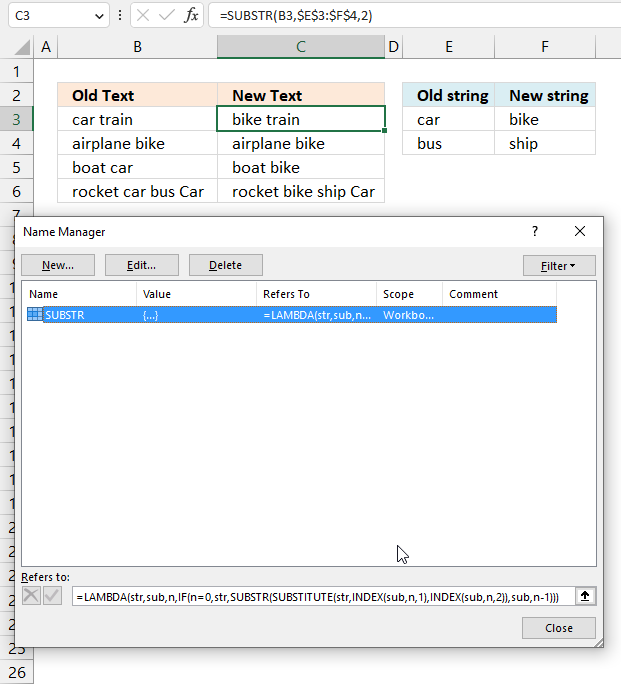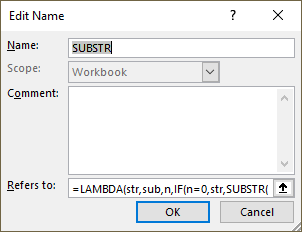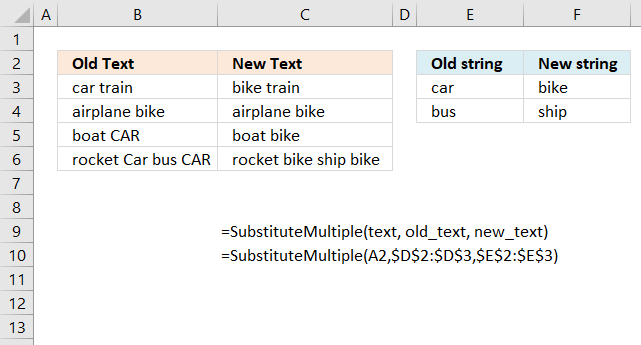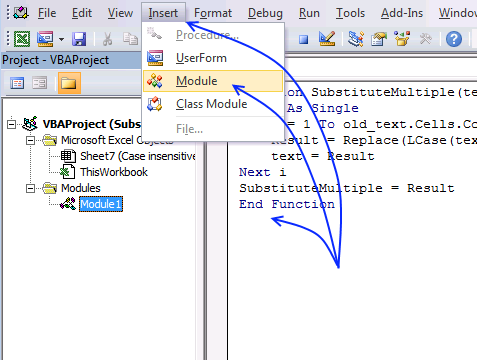Author: Oscar Cronquist Article last updated on March 06, 2023The SUBSTITUTE and REPLACE functions can only handle one string, this article demonstrates two ways to handle more than one pair of values.

• An Excel 365 recursive LAMBDA function.
• A User-Defined Function (UDF) allows you to substitute multiple text strings with new text strings.

## 1. Substitute multiple text strings - Excel 365 recursive LAMBDA functionThis example demonstrates a formula that iterates through all values in cell range E3:F4 in order to substitute specific values in cell B3 with new values.

The SUBSTITUTE function allows you to substitute one value with another value, however, this formula lets you substitute (almost) any number of values.

Excel 365 LAMBDA function in Name Manager:

=LAMBDA(str,sub,n,IF(n=0,str,SUBSTR(SUBSTITUTE(str,INDEX(sub,n,1),INDEX(sub,n,2)),sub,n-1)))

The LAMBDA function is named SUBSTR in the Name Manager.

Excel 365 formula in cell C3:

=SUBSTR(B3,\$E\$3:\$F\$4,2)

### 1.1 Explaining LAMBDA formula

#### Step 1 - Get old string

The INDEX function returns a value or reference from a cell range or array, you specify which value based on a row and column number.

Function syntax: INDEX(array, [row_num], [column_num])

INDEX(sub,n,1)

sub is a variable, in this example cell reference \$E\$3:\$F\$4

n is a number, representing the number of rows in cell reference \$E\$3:\$F\$4

1 is the first column in cell reference \$E\$3:\$F\$4

#### Step 2 - Get new string

The INDEX function returns a value or reference from a cell range or array, you specify which value based on a row and column number.

Function syntax: INDEX(array, [row_num], [column_num])

INDEX(sub,n,2)

sub is a variable, in this example cell reference \$E\$3:\$F\$4

n is a number, representing the number of rows in cell reference \$E\$3:\$F\$4

2 represents the second column in cell reference \$E\$3:\$F\$4

#### Step 3 - Substitute given values

The SUBSTITUTE function replaces a specific text string in a value. Case sensitive.

Function syntax: SUBSTITUTE(text, old_text, new_text, [instance_num])

SUBSTITUTE(str,INDEX(sub,n,1),INDEX(sub,n,2))

#### Step 4 - Make this formula recursive

The LAMBDA function is named SUBSTR in the name manager. We can call this named formula again until all values have been used.

SUBSTR(str,sub,n)

The SUBSTR function has three arguments:

• str - the string
• sub - the values to substitute
• n - the number of rows (or substitute pairs in cell ref \$E\$3:\$F\$4

SUBSTR(SUBSTITUTE(str,INDEX(sub,n,1),INDEX(sub,n,2)),sub,n-1)

str => SUBSTITUTE(str,INDEX(sub,n,1),INDEX(sub,n,2))

sub => sub (no change)

n => n - 1

n is keeping track of the row number. By subtracting one for each iteration the changes and a new pair of substitute values are processed.

#### Step 5 - Control the recursive formula

The IF function returns one value if the logical test is TRUE and another value if the logical test is FALSE.

Function syntax: IF(logical_test, [value_if_true], [value_if_false])

The IF function returns the string str if n is equal to 0 (zero), however, it calls the SUBSTR function again if not. This step is repeated like a loop until n is equal to 0 (zero).

IF(n=0,str,SUBSTR(SUBSTITUTE(str,INDEX(sub,n,1),INDEX(sub,n,2)),sub,n-1))

#### Step 6 - Create the LAMBDA function and define the parameters

The LAMBDA function build custom functions without VBA, macros or javascript.

Function syntax: LAMBDA([parameter1, parameter2, …,] calculation)

LAMBDA(str,sub,n,IF(n=0,str,SUBSTR(SUBSTITUTE(str,INDEX(sub,n,1),INDEX(sub,n,2)),sub,n-1)))

### 1.2 Name LAMBDA function in the Name Manager1. Go to tab "Formulas" on the ribbon.
2. Press the left mouse button on the "Name Manager" button.
A dialog box opens.
3. Press with left mouse button on the "New..." button. A new dialog box appears.
4. Name the function.5. Paste the LAMBDA formula in the "Refers to:" field.
6. Press with left mouse button on OK button.

You are now ready to use the named LAMBDA function. Select an empty cell. Type =SUBSTR( and the arguments, don't forget the ending parentheses. Press Enter.

### Get the Excel fileSubstitute-existing-text-with-multiple-text-strings-in-a-cell-range1.xlsx

## 2. How to use the User Defined FunctionYou may have as many strings as you like, there is really no limit. The image above shows the UDF in cell C3 using strings from E3:E4 and F3:F4.

Formula in cell C3:

=SubstituteMultiple(A2,\$D\$2:\$D\$3,\$E\$2:\$E\$3)

The UDF will not appear and work yet until you have copied the code below to a module in your workbook. There are instructions below.

Got it working? Now copy cell C3 and paste to cells below, the first argument contains relative cell references meaning they will change automatically when you copy and paste cell C3 to cells below.

The second and third argument are absolute cell references, they contain dollar signs meaning they are locked to cell range \$D\$2:\$D\$3 and \$E\$2:\$E\$3. These cell references will not change when you copy cell C3 and paste to the cells below.

### 2.1 User Defined Syntax

SubstituteMultiple(text As String, old_text As Range, new_text As Range)

### 2.2 Arguments

 text Required. A cell reference to a cell containing the text you want to manipulate. old_text Required. A cell reference to one or many cells containing strings you want to replace. new_text Required. A cell reference to one or many cells containing strings you want instead.

### 2.3 VBA code

```'Name function and dimension argument variables and declare their data types
Function SubstituteMultiple(text As String, old_text As Range, new_text As Range)

'Dimension variable and declare data type
Dim i As Single

'Iterate through cells in argument old_text
For i = 1 To old_text.Cells.Count

'Replace strings in value based on variable i
Result = Replace(LCase(text), LCase(old_text.Cells(i)), LCase(new_text.Cells(i)))

'Save manipulated value to variable text
text = Result
Next i

'Return value stored in variable Result to worksheet
SubstituteMultiple = Result
End Function
```

### 2.4 Where to put the code?1. Copy above VBA code.
2. Go to the Visual Basic Editor (Shortcut keys Alt + F11).
3. Press with left mouse button on Insert on the top menu.
4. Press with left mouse button on Module to create a module in your workbook. A module named module1 appears in the Project Explorer.
5. Paste code to module.
6. Exit VB Editor